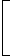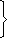# compound interest

6. What will be the compound interest on a sum of Rs. 25,000 after 3 years at the rate of 12 p.c.p.a.?
 A. Rs. 9000.30 B. Rs. 9720 C. Rs. 10123.20 D. Rs. 10483.20 E. None of these

Explanation:

Amount
 = Rs.25000 x1 + 123100
 = Rs.25000 x 28 x 28 x 2825 25 25
= Rs. 35123.20C.I. = Rs. (35123.20 – 25000) = Rs. 10123.20

7. At what rate of compound interest per annum will a sum of Rs. 1200 become Rs. 1348.32 in 2 years?
 A. 6% B. 6.5% C. 7% D. 7.5%

Explanation:

Let the rate be R% p.a.

 Then, 1200 x1 + R2 = 1348.32 1001 + R2 = 134832 = 11236 100 120000 100001 + R2 =1062 100 1001 + R = 106 100 100R = 6%

8. The least number of complete years in which a sum of money put out at 20% compound interest will be more than doubled is:
 A. 3 B. 4 C. 5 D. 6

Explanation:

 P1 + 20n > 2P6n > 2. 100 5

 Now,6 x 6 x 6 x 6> 2. 5 5 5 5

So, n = 4 years.

9. Albert invested an amount of Rs. 8000 in a fixed deposit scheme for 2 years at compound interest rate 5 p.c.p.a. How much amount will Albert get on maturity of the fixed deposit?
 A. Rs. 8600 B. Rs. 8620 C. Rs. 8820 D. None of these

Explanation:

Amount
 = Rs.8000 x1 + 52100
 = Rs.8000 x 21 x 2120 20
= Rs. 8820.

10. The effective annual rate of interest corresponding to a nominal rate of 6% per annum payable half-yearly is:
 A. 6.06% B. 6.07% C. 6.08% D. 6.09%

 Amount of Rs. 100 for 1 year when compounded half-yearly= Rs.100 x1 + 32= Rs. 106.09 100Effective rate = (106.09 – 100)% = 6.09%